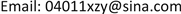1. 引言

2. 泥浆脉冲信号噪声干扰

3. 形态学滤波3.1. 形态学运算

f(n)关于g(m)腐蚀的关系式如下：

( f Θ g ) ( n ) = min { f ( n + m ) − g ( m ) } ， n = 0 , 1 , ⋯ , N − M (1)

f(n)关于g(m)膨胀的关系式如下：

( f ⊕ g ) ( n ) = max { f ( n − m ) + g ( m ) } ， n = 0 , 1 , ⋯ , N + M − 2 (2)

( f ∘ g ) ( n ) = ( f Θ g ⊕ g ) ( n ) (3)

( f • g ) ( n ) = ( f ⊕ g Θ g ) ( n ) (4)

3.2. 形态学滤波器设计

f 1 = 1 2 ( f 0 ∘ g 1 • g 1 + f 0 • g 1 ∘ g 1 ) (5)

f 2 = 1 2 ( f 1 ∘ g 2 • g 2 + f 1 • g 2 ∘ g 2 ) (5)

f 3 = f 0 − f 2 (6)

4. 试验分析

4.1. 试验样本

4.2. 结果分析4.2.1. 定性分析

4.2.2. 定量分析

The comparison of modified results of strong nonlinear baseline drift at high duty cycle of analog signal

The evaluation of modified results of strong nonlinear baseline drift at low duty cycle of analog signal

5. 结论

 梁耀. 随钻泥浆脉冲信号的处理[D]: [硕士学位论文]. 西安: 西安石油大学, 2013: 21-37.

 邱彬, 周静, 高建邦, 等. 随钻测量信号中泵冲噪声对消[J]. 石油机械, 2014, 42(12): 22-27.

 陈伟, 李晓林, 江佩佩. 基于2D-DCT变换的MWD遥传信号泵冲干扰消除方法[J]. 测控技术, 2016, 35(10): 19-22.

 廖琪梅, 李安宗, 屈景辉, 等. 随钻测井钻井液脉冲信号基线漂移的矫正[J]. 石油钻采工艺, 2008, 30(4): 41-43.

 郭晓鑫, 刘立, 冯志鹏. 数学形态学在信号处理中的应用综述[J]. 仪器仪表学报, 2009, 30(10): 187-191.

 刘艳丽, 赵为松, 李海坤, 等. 基于形态滤波的脉搏波信号基线漂移消除方法研究[J]. 合肥工业大学学报(自然科学版), 2011, 34(4): 525-528.

 庞宇, 邓璐, 林金朝, 等. 基于形态滤波的心电信号去除基线漂移方法[J]. 物理学报, 2014, 63(9): 1-5.

[编辑] 帅群

References梁耀. 随钻泥浆脉冲信号的处理[D]: [硕士学位论文]. 西安: 西安石油大学, 2013: 21-37.邱彬, 周静, 高建邦, 等. 随钻测量信号中泵冲噪声对消[J]. 石油机械, 2014, 42(12): 22-27.陈伟, 李晓林, 江佩佩. 基于2D-DCT变换的MWD遥传信号泵冲干扰消除方法[J]. 测控技术, 2016, 35(10): 19-22.廖琪梅, 李安宗, 屈景辉, 等. 随钻测井钻井液脉冲信号基线漂移的矫正[J]. 石油钻采工艺, 2008, 30(4): 41-43.郭晓鑫, 刘立, 冯志鹏. 数学形态学在信号处理中的应用综述[J]. 仪器仪表学报, 2009, 30(10): 187-191.刘艳丽, 赵为松, 李海坤, 等. 基于形态滤波的脉搏波信号基线漂移消除方法研究[J]. 合肥工业大学学报(自然科学版), 2011, 34(4): 525-528.庞宇, 邓璐, 林金朝, 等. 基于形态滤波的心电信号去除基线漂移方法[J]. 物理学报, 2014, 63(9): 1-5.